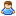1.You are currently browsing our forum as a guest. Create your own forum account to access all forum functionality.

# user defined arrays

Discussion in 'Programming Questions and Suggestions' started by Dengue, Feb 9, 2015.

This last post in this thread was made more than 31 days old.
1. ### DengueTrainee Engineer

Messages:
17
hi,
I am writing this here cause I need a help with arrays.
This is what I wrote as code for 2 dimensional array attribution and sorting.
Code:
```Main
{
List&lt;IMyTerminalBlock> rotor= new List&lt;IMyTerminalBlock>();
GridTerminalSystem.GetBlocksOfType&lt;IMyMotorStator>(rotor);
int[,] vet = new int[rotor.Count,3];
int [,] vet2= new int[1,3];
int aux=0,i=0,j=0,k=0;
int pri=0, ult=0;
string sub="";
for(i=0;i&lt;rotor.Count;i++)
{
for(j=0;j&lt;3;j++)
{
if(rotor[i].GetPosition().ToString().IndexOf(" ")!=-1)
{
pri=rotor[i].GetPosition().ToString().IndexOf(":");
ult=rotor[i].GetPosition().ToString().IndexOf(" ");
vet[i,j]=int.Parse(rotor[i].GetPosition().ToString().Substring(pri,ult));
}
}
}
for(i=0;i&lt;rotor.Count;i++)
{
for(j=0;j&lt;3;j++)
{
vet2[i,j]=i;
}
}
for(k=0;k&lt;3;k++)
{
for(i=1;i&lt;rotor.Count;i++)
{
for(j=rotor.Count-1;j>=i;j--)
{
if(vet[j-1,k]>vet[j,k])
{
aux=vet2[j-1,k];
vet2[j-1,k]=vet2[j,k];
vet2[j,k]=aux;
}
}
}
}
}
```
about the code, I get a Type.System.Int32 not allowed in scripts.
another note, this code gets the value of position and put in an array,
then it creates another array , load and sort this array values.
at the end I will name my rotors the second array values to indicate what is the order of wich rotor in position, getting the first as the highest and the last as the lowest, so I can name my rotors with the name of its specifical area and function.

I used the bubble method mixed with another array for sorting.
the thing is, how to write 2 dimensional array with user defined values like block.GetPosition() or anything else, I already readed about lists and such, but I see only examples of people getting blocks to make actions and/or count blocks.
without normal array I cant see a solution.

Last edited by a moderator: Feb 9, 2015
2. ### Igneous01Apprentice Engineer

Messages:
266
I'm not sure I entirely understand (plus the formatting came out bad for your post).
But are you trying to store custom types into a 2d array?

If thats the case most efficient way would be to create a struct and store this in the array.

Code:
`Array&lt;Array&lt;myStruct>> multi_array;`
Then just set up your struct to store whatever members you may need to reference later.

If you're asking how to store two different types, then its better to use a dictionary or map.

Code:
`Dictionary&lt;myStruct,int> map;`
This is assuming I understood your question correctly.

3. ### DengueTrainee Engineer

Messages:
17
thanks for your answer and nice to know that 2 dimensional arrays are possible.
Now I come with another question,
how to access positions of this new array?
I tried doing this way:
Code:
```//creates an array
int vector[][] = new int;
//define a value to array
vector=1;
```
it didnt work because of the double "[]".

how to make this work?
Code:
```array&lt;array&lt;MyStruct>> array01;//what is the size of the array?
array01=1;//may get a problem because of the two indexes

```

4. ### ImmersiveApprentice Engineer

Messages:
122
You must instantiate each dimension individually, IIRC, because they are actually arrays of arrays.
Code:
``` int vector[][] = new int { new int, new int } };
int vector[][] = new int { {0,0,0}, {0,0,0} };
```
That said, your code looks awkward. Would you not be better served using arrays of Vectors?

Last edited by a moderator: Feb 10, 2015
5. ### DengueTrainee Engineer

Messages:
17
I dont get you.
You said me to do two arrays of three elements, as I can see they work separately.

let me clear how a 2 dimensional array works.
Code:
```int[] vector=new int; //create and declare an array
//let me load all array positions manually
vector=1;
vector=1;
vector=1;
vector=2;
vector=2;
vector=2;
```
as you can see every position of the first index works as a new position for all the second indexes too.

your concept of array is wrong.

6. ### ImmersiveApprentice Engineer

Messages:
122
Let me assure you I am very familiar with how multi-dim arrays work. Perhaps your use of them is wrong?

Ed: removed my rant, which was mostly wrong.

MSDN C# Multidimensional Arrays (top result for googling "C# multidimensional array")

Keep in mind that Keen has mangled some of the language features in their efforts to hack-proof the script engine, and multi-dim arrays may be one of those features.

Please try my suggestion. If it doesn't work then we can try something else.

Last edited by a moderator: Feb 11, 2015